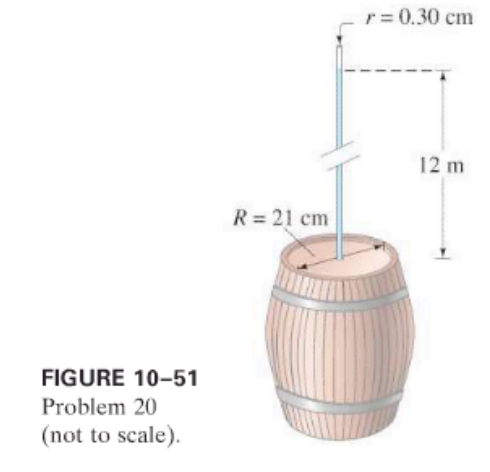×
Get Full Access to Physics: Principles With Applications - 6 Edition - Chapter 10 - Problem 20p
Get Full Access to Physics: Principles With Applications - 6 Edition - Chapter 10 - Problem 20p

×

# In working out his principle, Pascal showed dramaticallyISBN: 9780130606204 3

## Solution for problem 20P Chapter 10

Physics: Principles with Applications | 6th Edition

• Textbook Solutions
• 2901 Step-by-step solutions solved by professors and subject experts
• Get 24/7 help from StudySoup virtual teaching assistantsPhysics: Principles with Applications | 6th Edition

4 5 1 338 Reviews
24
4
Problem 20P

In working out his principle, Pascal showed dramatically how force can be multiplied with fluid pressure. He placed a long, thin tube of radius $$r=0.30 \mathrm{~cm}$$ vertically into a wine barrel of radius $$R=21 \mathrm{~cm}$$, Fig. 10-51. He found that when the barrel was filled with water and the tube filled to a height of 12 m, the barrel burst. Calculate

(a) the mass of water in the tube, and

(b) the net force exerted by the water in the barrel on the lid just before rupture.Equation Transcription:Text Transcription:

r=0.30 cm

R=21 cm

Step-by-Step Solution:

Solution 20P:

We have to calculate the mass of the water inside the tube and the force exerted by the water in the barrel on the lid just before rupture.

Step 1 of 3

Concept:

The ratio of the massof a substance to its volumeis always constant, and it is known as the densityof the substance. Mathematically,…(1)

Pressureon the body is defined as the forceapplied per unit cross-sectional areaof the body. Mathematically,…(2)

Pressuredue to liquid of densityhaving its column height, is given as…(3)

Step 2 of 3

Step 3 of 3

##### ISBN: 9780130606204

Physics: Principles with Applications was written by and is associated to the ISBN: 9780130606204. This textbook survival guide was created for the textbook: Physics: Principles with Applications, edition: 6. Since the solution to 20P from 10 chapter was answered, more than 1104 students have viewed the full step-by-step answer. The answer to “?In working out his principle, Pascal showed dramatically how force can be multiplied with fluid pressure. He placed a long, thin tube of radius $$r=0.30 \mathrm{~cm}$$ vertically into a wine barrel of radius $$R=21 \mathrm{~cm}$$, Fig. 10-51. He found that when the barrel was filled with water and the tube filled to a height of 12 m, the barrel burst. Calculate (a) the mass of water in the tube, and (b) the net force exerted by the water in the barrel on the lid just before rupture. Equation Transcription: Text Transcription:r=0.30 cm R=21 cm” is broken down into a number of easy to follow steps, and 95 words. This full solution covers the following key subjects: barrel, Water, tube, radius, Force. This expansive textbook survival guide covers 35 chapters, and 3914 solutions. The full step-by-step solution to problem: 20P from chapter: 10 was answered by , our top Physics solution expert on 03/03/17, 03:53PM.

## Discover and learn what students are asking

Unlock Textbook Solution